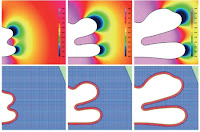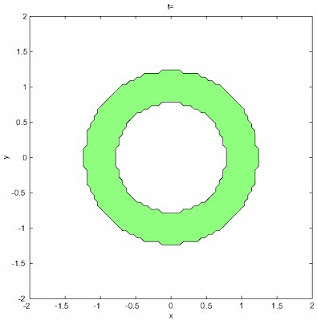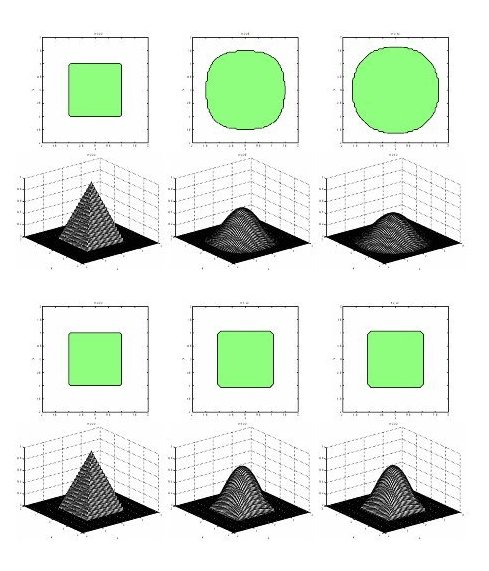### Relaxation approximations and numerical solution of degenerate diffusion equationsHele-Shaw cell
Nonlinear diffusion equations come from a variety of diffusion phenomena which appear widely in nature. They are suggested as mathematical models of physical problems in many fields, such as filtration, phase transition, biochemistry, thin films and dynamics of biological groups. In many cases, the equations possess degeneracy or singularity. The appearance of degeneracy or singularity makes the development of numerical schemes more involved and challenging.
In [1, 2] we presented a class of relaxation schemes for second and fourth order degenerate diffusion equations. For second order degenerate diffusion (or convection-diffusion) equations the schemes are based on the introduction of a suitable relaxation approximation that permits to reduce the second order equations to first order semi-linear hyperbolic systems with stiff terms. In a similar way we have shown how fourth order diffusion equations can be approximated by second or first order relaxation systems with stiff terms. The numerical passage from the relaxation system to the nonlinear diffusion equation is realized by using suitable semi-implicit or fully implicit time discretizations combined with upwind and central differences in space. Some numerical results and simple animations of  porous media and thin films equations in  two space dimensions are reported here. See also  for a related numerical approach to fourth order problems based on probabilistic methods.

Two dimensional porous media with absorptionTwo dimensional thin films equationEvolution of the interface front for the two dimensional thin films equation with exponent m=1 (spreading of the support, top) and m=4 (no spreading of the support, bottom)

References
1. G.Naldi, L.Pareschi, G.Toscani, Hyperbolic relaxation approximation to nonlinear parabolic problems, Proceedings 7th Int. Conf. on Hyperbolic Problems: Theory, Numerics, Application, ETH Zurich 1998, Internat. Series of Num. Math., Vol. 130, Birkhauser Verlag Basel, pp. 747-756, (1999).
2. G.Naldi, L.Pareschi, G.Toscani, Relaxation schemes for PDEs and applications to fourth order diffusion equations, Surveys on Mathematics for Industry, 10, pp.315-343, (2002).
3. L.Pareschi, G.Russo, G.Toscani, A kinetic approximation to Hele-Shaw flow, C. R., Math., Acad. Sci. Paris, Ser. I 338, No.2, pp.177-182 (2004).